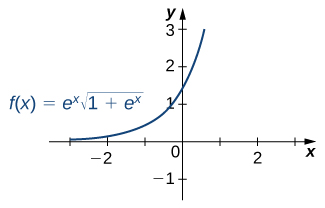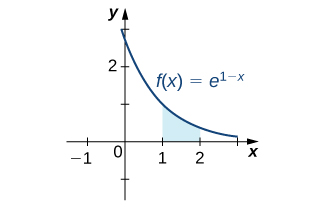# Integrals of Exponential Functions

Integrating Exponential Functions By Substitution – Antiderivatives – Calculus
Integrating Exponential Functions By Substitution – Antiderivatives – Calculus

### Learning Outcomes

• Integrate functions involving exponential functions

The exponential function is perhaps the most efficient function in terms of the operations of calculus. The exponential function, $y={e}^{x},$ is its own derivative and its own integral.

### Integrals of Exponential Functions

Exponential functions can be integrated using the following formulas.

The nature of the antiderivative of ${e}^{x}$ makes it fairly easy to identify what to choose as $u$. If only one $e$ exists, choose the exponent of $e$ as $u$. If more than one $e$ exists, choose the more complicated function involving $e$ as $u$.

### Example: Finding an Antiderivative of an Exponential Function

Find the antiderivative of the exponential function $e^{-x}$.

Use substitution, setting $u=\text{−}x,$ and then $du=-1dx.$ Multiply the du equation by −1, so you now have $\text{−}du=dx.$ Then,

$\begin{array}{cc}{\displaystyle\int {e}^{\text{−}x}dx}\hfill & =\text{−}{\displaystyle\int {e}^{u}du}\hfill \\ \\ & =\text{−}{e}^{u}+C\hfill \\ & =\text{−}{e}^{\text{−}x}+C.\hfill \end{array}$

### Try It

Find the antiderivative of the function using substitution: ${x}^{2}{e}^{-2{x}^{3}}.$

Let $u$ equal the exponent on $e$.

$\displaystyle\int {x}^{2}{e}^{-2{x}^{3}}dx=-\frac{1}{6}{e}^{-2{x}^{3}}+C$

Watch the following video to see the worked solution to the above Try It.

You can view the transcript for this segmented clip of “5.6.1” here (opens in new window).

A common mistake when dealing with exponential expressions is treating the exponent on $e$ the same way we treat exponents in polynomial expressions. We cannot use the power rule for the exponent on $e$. This can be especially confusing when we have both exponentials and polynomials in the same expression, as in the previous checkpoint. In these cases, we should always double-check to make sure we’re using the right rules for the functions we’re integrating.

### Example: Square Root of an Exponential Function

Find the antiderivative of the exponential function ${e}^{x}\sqrt{1+{e}^{x}}.$

First rewrite the problem using a rational exponent:

Using substitution, choose $u=1+{e}^{x}.u=1+{e}^{x}.$ Then, $du={e}^{x}dx.$ We have (Figure 1)

ThenFigure 1. The graph shows an exponential function times the square root of an exponential function.

### Try It

Find the antiderivative of ${e}^{x}{(3{e}^{x}-2)}^{2}.$

Let $u=3{e}^{x}-2u=3{e}^{x}-2.$

$\displaystyle\int {e}^{x}{(3{e}^{x}-2)}^{2}dx=\frac{1}{9}{(3{e}^{x}-2)}^{3}$

Watch the following video to see the worked solution to the above Try It.

You can view the transcript for this segmented clip of “5.6.1” here (opens in new window).

### Example: Using Substitution with an Exponential Function

Use substitution to evaluate the indefinite integral $\displaystyle\int 3{x}^{2}{e}^{2{x}^{3}}dx.$

Here we choose to let $u$ equal the expression in the exponent on $e$. Let $u=2{x}^{3}$ and $du=6{x}^{2}dx..$ Again, du is off by a constant multiplier; the original function contains a factor of 3$x$2, not 6$x$2. Multiply both sides of the equation by $\frac{1}{2}$ so that the integrand in $u$ equals the integrand in $x$. Thus,

Integrate the expression in $u$ and then substitute the original expression in $x$ back into the $u$ integral:

### Try It

Evaluate the indefinite integral $\displaystyle\int 2{x}^{3}{e}^{{x}^{4}}dx.$

Let $u={x}^{4}.$

$\displaystyle\int 2{x}^{3}{e}^{{x}^{4}}dx=\frac{1}{2}{e}^{{x}^{4}}$

As mentioned at the beginning of this section, exponential functions are used in many real-life applications. The number e is often associated with compounded or accelerating growth, as we have seen in earlier sections about the derivative. Although the derivative represents a rate of change or a growth rate, the integral represents the total change or the total growth. Let’s look at an example in which integration of an exponential function solves a common business application.

A price–demand function tells us the relationship between the quantity of a product demanded and the price of the product. In general, price decreases as quantity demanded increases. The marginal price–demand function is the derivative of the price–demand function and it tells us how fast the price changes at a given level of production. These functions are used in business to determine the price–elasticity of demand, and to help companies determine whether changing production levels would be profitable.

### Example: Finding a Price–Demand Equation

Find the price–demand equation for a particular brand of toothpaste at a supermarket chain when the demand is 50 tubes per week at $2.35 per tube, given that the marginal price—demand function, ${p}^{\prime }(x),$ for $x$ number of tubes per week, is given as If the supermarket chain sells 100 tubes per week, what price should it set? To find the price–demand equation, integrate the marginal price–demand function. First find the antiderivative, then look at the particulars. Thus, Using substitution, let $u=-0.01x$ and $du=-0.01dx.$ Then, divide both sides of the du equation by −0.01. This gives The next step is to solve for C. We know that when the price is$2.35 per tube, the demand is 50 tubes per week. This means

Now, just solve for C:

Thus,

If the supermarket sells 100 tubes of toothpaste per week, the price would be

The supermarket should charge \$1.99 per tube if it is selling 100 tubes per week.

Watch the following video to see the worked solution to Example: Finding a Price–Demand Equation.

You can view the transcript for this segmented clip of “5.6.1” here (opens in new window).

### example: Evaluating a Definite Integral Involving an Exponential Function

Evaluate the definite integral ${\displaystyle\int }_{1}^{2}{e}^{1-x}dx.$

Again, substitution is the method to use. Let $u=1-x,$ so $du=-1dx$ or $\text{−}du=dx.$ Then $\displaystyle\int {e}^{1-x}dx=\text{−}\displaystyle\int {e}^{u}du.$ Next, change the limits of integration. Using the equation $u=1-x,$ we have

The integral then becomes

See Figure 2.Figure 2. The indicated area can be calculated by evaluating a definite integral using substitution.

### Try It

Evaluate ${\displaystyle\int }_{0}^{2}{e}^{2x}dx.$

Let $u=2x$

$\frac{1}{2}{\displaystyle\int }_{0}^{4}{e}^{u}du=\frac{1}{2}({e}^{4}-1)$

### Example: Growth of Bacteria in a Culture

Suppose the rate of growth of bacteria in a Petri dish is given by $q(t)={3}^{t},$ where $t$ is given in hours and $q(t)$ is given in thousands of bacteria per hour. If a culture starts with 10,000 bacteria, find a function $Q(t)$ that gives the number of bacteria in the Petri dish at any time $t$. How many bacteria are in the dish after 2 hours?

We have

$Q(t)=\int {3}^{t}dt=\frac{{3}^{t}}{\text{ln}3}+C.$

Then, at $t=0$ we have $Q(0)=10=\frac{1}{\text{ln}3}+C,$ so $C\approx 9.090$ and we get

$Q(t)=\frac{{3}^{t}}{\text{ln}3}+9.090.$

At time $t=2,$ we have

After 2 hours, there are 17,282 bacteria in the dish.

### Try It

From (Figure), suppose the bacteria grow at a rate of $q(t)={2}^{t}.$ Assume the culture still starts with 10,000 bacteria. Find $Q(t).$ How many bacteria are in the dish after 3 hours?

Use the procedure from (Figure) to solve the problem.

$Q(t)=\frac{{2}^{t}}{\text{ln}2}+8.557.$ There are 20,099 bacteria in the dish after 3 hours.

### Fruit Fly Population Growth

Suppose a population of fruit flies increases at a rate of $g(t)=2{e}^{0.02t},$ in flies per day. If the initial population of fruit flies is 100 flies, how many flies are in the population after 10 days?

Let $G(t)$ represent the number of flies in the population at time $t$. Applying the net change theorem, we have

There are 122 flies in the population after 10 days.

### Try It

Suppose the rate of growth of the fly population is given by $g(t)={e}^{0.01t},$ and the initial fly population is 100 flies. How many flies are in the population after 15 days?

### example: Evaluating a Definite Integral Using Substitution

Evaluate the definite integral using substitution: ${\displaystyle\int }_{1}^{2}\dfrac{{e}^{1\text{/}x}}{{x}^{2}}dx.$

This problem requires some rewriting to simplify applying the properties. First, rewrite the exponent on $e$ as a power of $x$, then bring the $x$2 in the denominator up to the numerator using a negative exponent. We have

Let $u={x}^{-1},$ the exponent on $e$. Then

Bringing the negative sign outside the integral sign, the problem now reads

Next, change the limits of integration:

Notice that now the limits begin with the larger number, meaning we must multiply by −1 and interchange the limits. Thus,

### Try It

Evaluate the definite integral using substitution: ${\displaystyle\int }_{1}^{2}\frac{1}{{x}^{3}}{e}^{4{x}^{-2}}dx.$

Let $u=4{x}^{-2}$

${\displaystyle\int }_{1}^{2}\frac{1}{{x}^{3}}{e}^{4{x}^{-2}}dx=\frac{1}{8}\left[{e}^{4}-e\right]$

You are watching: Integrals of Exponential Functions. Info created by THVinhTuy selection and synthesis along with other related topics.

Rate this post